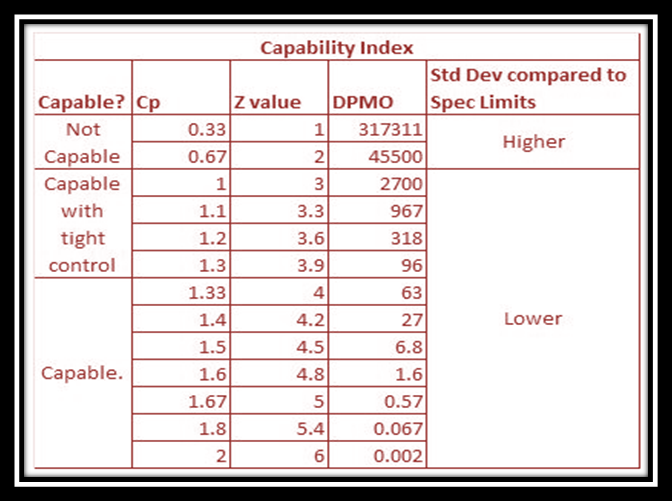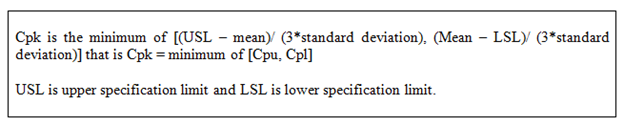# Process Capability Formula with Examplesby StarAgile

September 02, 2020
Category Six Sigma

The development of any product or service must follow a well-structured process and the ability of this course of action will decide the successful outcome. This is satisfied if the process designed matches the customer need.

Before we move forward let us begin with an example to provide you with a sign of relief that we can achieve at the right process with simple math.

## Process Capability Example 1: The ice cream that must be served in an ice cream parlor has to be between -15 degrees Celsius and -35 degrees Celsius. The process of refrigeration that keeps the temperature has a standard deviation (SD) of 2 degrees Celsius. And the mean value of this temperature is -25 degrees Celsius. Using these inputs obtain the process capability index  (PCI) for this process?

Note – In this section do not bother about any technical terms just understand how to use the formula. Later you will find details.

USL = -15

LSL = -35

SD = 2,

Mean = -25

## Formula

Cpu = Mean – LSL / 3 X SD

Cpl = USL – Mean / (3 X SD)

Cpk  = Min (Cpu, Cpl)

Substitute the values in the formula

Cpu = (-15-(-25))/ (3*2) = -10/6 = -1.667

Cpl = (-25+35)/ (3*2) = 10/6 = 1.667

We know that Cpk is a minimum of [Cpu, Cpl] and hence a minimum of [-1.667, 1.667] is -1.667. Therefore the upper limit seems to suit best for this condition.

In the following section, you will find another example with a detailed explanation of each terminology used.

## What is Process Capability?

It refers to the arithmetical study of the natural process variability of the given responsibilities in consideration.

Cp – This is how it is represented. It denotes the capability of the process that can be achieved when perfectly centered between the lower and the upper limit.

Cpk – This is also used for calculating the ability of the process; it determines how close you are to the given target and how consistent you are around your average performance.

Another way to study the ability of the performance is by finding the Pp and the Ppk.

• The Cp and Cpk are used for processes that are old, matured, and are under control statistically. Whereas the Pp and Ppk are used for the processes that are new and are not possible to determine their statistical control factor.

In this article, we will discuss Cp and Cpk is a process capability study.

Also, if you want to improve the process capability, then you need to apply the right design thinking which is discussed in DFSS (design for six sigma).

## Process capability Formula and Calculation

The Cp has 2 subdivisions

1. Cp upper that is Cpu
2. Cp lower that is Cpl

These can be calculated with the formula

Cpu = (Process mean - LSL)/ (3 * Standard deviation) where the LSL is the lower specification limit.

Cpl = (USL  - Process Mean)/ (3 * Standard deviation) where the USL is the upper specification limit.

Now the term Cpk is only the value that is smallest of the above two, that is Cpu and Cpl.## Process Capability Index Formula

Cpk is the smallest of the Cpu and Cpl. Let’s take an example

Example 2: - The training room temperature during the training must be kept between 18 degrees Celsius and 26 degrees Celsius. The process of maintaining this temperature has a standard deviation of 2 degrees Celsius. If the mean temperature is 21 degrees Celsius, find the PCI.Here USL is 26 and LSL is 18 and the Standard deviation is 2, and the mean is 21.

Cpu= (26 - 21)/ (3 * 2) = 5/6 = 0.8334

Cpl = (21 - 18)/ (3 * 2) = 3/6 = 0.5

Cpk = Minimum of [ Cpu, Cpl]

That is Cpk = minimum of [0.8334, 0.5]

The process capability index Cpk is 0.5

## The practical difficulty in conducting process capability study

• Cp and Cpk values will not be accurate if the processes are not stable, in this case, we need to use Process performance values such as Pp and Ppk.
• For a centered process the Cp value will give the correct estimate and for a not centered process, the Cpk value will be the correct value.
• Further sampling from the stable system is essential when obtaining meaningful estimates of the process performance for future production.
• Other stable and not normal distribution can be studied as a part of the comparison to normal distribution to obtain meaningful estimates.
• The processes must be under control analytically to obtain the correct value of Cp and Cpk otherwise it provides the wrong value.
• The processes on which you find the Cp and Cpk values must be matured enough and old.
• The value of Cp and Cpk are sensitive that it uses the sampling from the normal distribution and assumed that most of the data points are centered on the mean that is the bell-shaped curve.

## Process Capability Ratio

As discussed in the process capability example in the above paragraph we need to find out the inconsistency in the process with the help of the upper and lower limits respectively.

The following can be seen from the analysis and process capability ratio:

• When Cp equals 1, the process is perfectly centered. (Cp = 1, prefect process)
• For Cp value higher than one, it means tolerance> the process. This confirms that the process appears to be able to do this. (Cp> 1, process compatible)
• If the process length is greater than the tolerance, the process variation is within the tolerance range, rendering the process incapable.
• Cpk makes it possible to measure the closeness of the performance of the process to the condition lay down by the client by allowing for the natural inconsistency of the process.
• If Cpk is a negative value, you need to make sure that the process you inherited leads to a result that is not within the range specified by the client and is completely outside.
• The Cpk of 3 indicates that the output is excellent and the process has 6σ capacities and that is what we need to achieve.
• We need a Cpk of at least 1.33, which is 4σs, to satisfy most customers.

## Conclusion

6σ is a numerical study of deviations in the process. This is important because due to such variations the result of the product or service will also vary. Usually, that is the reason the customers provide the range (lower and upper) for any product that they can accept.

In a real sense, there is no perfect process and hence no perfect product as it is very difficult to meet perfection. That is why there are certain tolerances allowed. Thus to reduce the defects in the product the process must follow some criteria so that the products fall exactly or near the center of the sigma line defined by the upper and lower tolerance levels.

As 6σ is a statistical tool that determines sigma value and the process capability ratio and process capability analysis, you might be using this very often as a process champion at your organizations.

Now that you have a brief idea of the process capabilities and how to find the Cp and Cpk value using the formulae. To explore more on six sigma and its various tools we suggest you register for the online interactive six sigma green belt training with StarAgile. To register click on the link provided. Keep Learning!!!